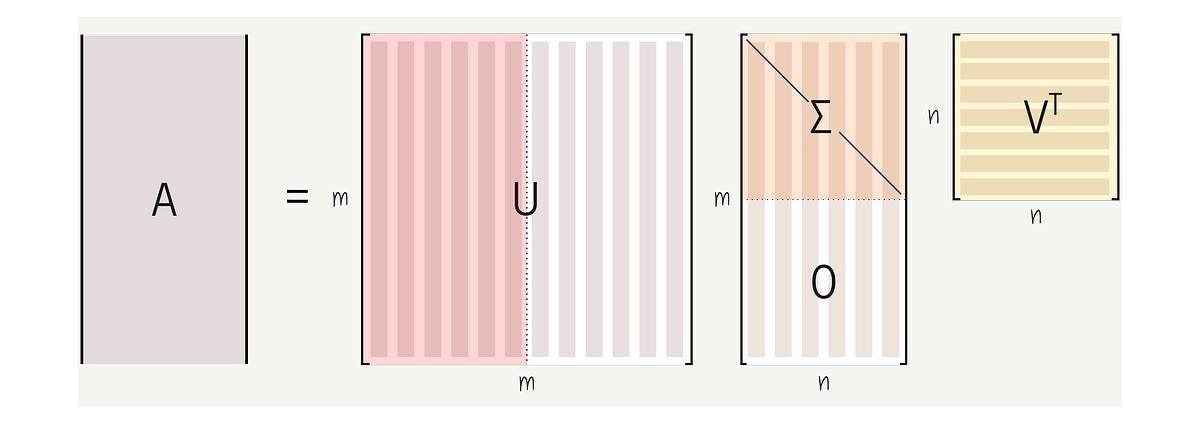# Understanding Linear Regression using the Singular Value DecompositionUnderstanding Linear Regression using the Singular Value Decomposition. In this post, we will also talk about solving linear regression problems but through a different perspective. Most specifically, we will talk about one of the most fundamental applications of linear algebra and how we can use it to solve regression problems.

## Introduction

It is very common to see blog posts and educational material explaining linear regression. In most cases, probably because of the big data and deep learning biases, most of these educational resources take the gradient descent approach to fit lines, planes, or hyperplanes to high dimensional data. In this post, we will also talk about solving linear regression problems but through a different perspective. Most specifically, we will talk about one of the most fundamental applications of linear algebra and how we can use it to solve regression problems. Yes, I am talking about the SVD or the Singular Value Decomposition. This computational tool is used as a basis to solve a myriad of problems, including dimensionality reduction, with PCA, and statistical learning using linear regression.

## Linear Models and Systems of Linear Equations

Through the lens of linear algebra, a regression problem reduces to solving systems of linear equations of the form Ax = b. Here,** A and **b are known, and x is the unknown. We can think of x as our model. In other words, we want to solve the system for x, and hence, x is the variable that relates the observations in A to the measures in b.

Here, A is a data matrix. We can think of the rows of A as representing different instances of the same phenomenon. They can represent records for individual patients submitted to a hospital, records for different houses being sold, or pictures of different people’s faces. Complementary, we can view the columns of the matrix A as recording different characteristics of each instance in the rows of A. In a patient hospital example, such features might include the blood pressure when he/she arrived at the hospital or if the patient has had a surgical procedure or not.

Also, note that the matrix A might have different shapes. First, A could be a square matrix. Yes, it is very unlikely (for the situations we usually encounter in data science) but otherwise possible.

## Data Science Projects | Data Science | Machine Learning | Python

Practice your skills in Data Science with Python, by learning and then trying all these hands-on, interactive projects, that I have posted for you.

## Data Science Projects | Data Science | Machine Learning | Python

Practice your skills in Data Science with Python, by learning and then trying all these hands-on, interactive projects, that I have posted for you.

## Data Science Projects | Data Science | Machine Learning | Python

Practice your skills in Data Science with Python, by learning and then trying all these hands-on, interactive projects, that I have posted for you.

## Data Science Projects | Data Science | Machine Learning | Python

Practice your skills in Data Science with Python, by learning and then trying all these hands-on, interactive projects, that I have posted for you.

## Data Science Projects | Data Science | Machine Learning | Python

Practice your skills in Data Science with Python, by learning and then trying all these hands-on, interactive projects, that I have posted for you.